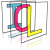Image Component Library (ICL)
icl::geom::Camera Class Reference

Camera class. More...

#include <Camera.h>

## Classes

struct  NotEnoughDataPointsException
We need at least 6 Data points in general positions. More...

struct  RenderParams

## Public Types

typedef math::FixedMatrix< icl32f, 3, 3 > Mat3x3
internal typedef More...

## Public Member Functions

utils::Point32f project (const Vec &Xw) const
Project a world point onto the image plane. Caution: Set last component of world points to 1. More...

void project (const std::vector< Vec > &Xws, std::vector< utils::Point32f > &dst) const
Project a vector of world points onto the image plane. Caution: Set last component of world points to 1. More...

const std::vector< utils::Point32fproject (const std::vector< Vec > &Xws) const
Project a vector of world points onto the image plane. Caution: Set last component of world points to 1. More...

Vec projectGL (const Vec &Xw) const
Project a world point onto the image plane. More...

void projectGL (const std::vector< Vec > &Xws, std::vector< Vec > &dst) const
Project a vector of world points onto the image plane. More...

const std::vector< VecprojectGL (const std::vector< Vec > &Xws) const
Project a vector of world points onto the image plane. More...

ViewRay getViewRay (const utils::Point32f &pixel) const
Returns a view-ray equation of given pixel location. More...

std::vector< ViewRaygetViewRays (const std::vector< utils::Point32f > &pixels) const
returns a list of viewrays corresponding to a given set of pixels More...

utils::Array2D< ViewRaygetAllViewRays () const
returns a 2D array of all viewrays More...

ViewRay getViewRay (const Vec &Xw) const
Returns a view-ray equation of given point in the world. More...

Vec estimate3DPosition (const utils::Point32f &pixel, const PlaneEquation &plane) const
returns estimated 3D point for given pixel and plane equation More...

void setRotation (const Mat3x3 &rot)
set the norm and up vectors according to the passed rotation matrix More...

void setRotation (const Vec &rot)
set norm and up vectors according to the passed yaw, pitch and roll More...

void setTransformation (const Mat &m)
sets the camera's rotation and position from given 4x4 homogeneous matrix More...

void setWorldTransformation (const Mat &m)
this sets the camera to the given world tranformation More...

void setWorldFrame (const Mat &m)
adapts the camera transformation, so that the given frame becomes the world frame More...

Mat getCSTransformationMatrix () const
get world to image coordinate system transformation matrix More...

Mat getInvCSTransformationMatrix () const
returns the transform of the camera wrt. world frame More...

Mat getCSTransformationMatrixGL () const
get world to image coordinate system transformation matrix More...

Mat getProjectionMatrix () const
get projection matrix More...

Mat getProjectionMatrixGL () const
get the projection matrix as expected by OpenGL More...

Mat getViewportMatrixGL () const

math::FixedMatrix< icl32f, 4, 3 > getQMatrix () const
returns the common 4x3 camera matrix More...

math::FixedMatrix< icl32f, 3, 4 > getInvQMatrix () const
returns the inverse QMatrix More...

void translate (const Vec &d)
translates the current position vector More...

std::string toString () const

const std::string & getName () const

const VecgetPosition () const

const VecgetNorm () const

const VecgetUp () const

Vec getHoriz () const

float getFocalLength () const

utils::Point32f getPrincipalPointOffset () const

float getPrincipalPointOffsetX () const

float getPrincipalPointOffsetY () const

float getSamplingResolutionX () const

float getSamplingResolutionY () const

float getSkew () const

const RenderParamsgetRenderParams () const

RenderParamsgetRenderParams ()

void setName (const std::string &name)

void setPosition (const Vec &pos)

void setNorm (const Vec &norm, bool autoOrthogonalizeRotationMatrix=false)

void setUp (const Vec &up, bool autoOrthogonalizeRotationMatrix=false)
gets automatically normalized More...

void orthogonalizeRotationMatrix ()
extracts the current rotation matrix and uses gramSchmidth orthogonalization More...

void setFocalLength (float value)

void setPrincipalPointOffset (float px, float py)

void setPrincipalPointOffset (const utils::Point32f &p)

void setSamplingResolutionX (float value)

void setSamplingResolutionY (float value)

void setSamplingResolution (float x, float y)

void setSkew (float value)

void setRenderParams (const RenderParams &rp)

void setResolution (const utils::Size &newScreenSize)
Changes the camera resolution and adapts dependent values. More...

void setResolution (const utils::Size &newScreenSize, const utils::Point &newPrincipalPointOffset)
Changes the camera resolution and adapts dependent values. More...

const utils::SizegetResolution () const
returns the current chipSize (camera resolution in pixels) More...

Mat estimatePose (const std::vector< Vec > &objectCoords, const std::vector< utils::Point32f > &imageCoords, bool performLMAOptimization=true)
estimate world frame pose of object specified by given object points More...

## Static Public Member Functions

static Camera createFromProjectionMatrix (const math::FixedMatrix< icl32f, 4, 3 > &Q, float focalLength=1)
Compute all camera parameters from the 4x3 projection matrix. More...

static Camera calibrate (std::vector< Vec > Xws, std::vector< utils::Point32f > xis, float focalLength=1, bool performLMAOptimization=true)
Uses the passed world point – image point references to estimate the projection parameters. More...

static Camera calibrate_pinv (std::vector< Vec > Xws, std::vector< utils::Point32f > xis, float focalLength=1, bool performLMAOptimization=true)
Uses the passed world point – image point references to estimate the projection parameters. More...

static Camera calibrate_extrinsic (const std::vector< Vec > &Xws, const std::vector< utils::Point32f > &xis, const Camera &intrinsicCamValue, const RenderParams &renderParams=RenderParams(), bool performLMAOptimization=true)
performs extrinsic camera calibration using a given set of 2D-3D correspondences and the given intrinsic camera calibration data More...

static Camera calibrate_extrinsic (const std::vector< Vec > &Xws, const std::vector< utils::Point32f > &xis, const Mat &camIntrinsicProjectionMatrix, const RenderParams &renderParams=RenderParams(), bool performLMAOptimization=true)
performs extrinsic camera calibration using a given set of 2D-3D correspondences and the given intrinsic camera calibration data More...

static Camera calibrate_extrinsic (std::vector< Vec > Xws, std::vector< utils::Point32f > xis, float fx, float fy, float s, float px, float py, const RenderParams &renderParams=RenderParams(), bool performLMAOptimization=true)
performs extrinsic camera calibration using a given set of 2D-3D correspondences and the given intrinsic camera calibration data More...

static Camera optimize_camera_calibration_lma (const std::vector< Vec > &Xws, const std::vector< utils::Point32f > xis, const Camera &init)
performs a non-linear LMA-based optimization to improve camera calibration results More...

static Vec getIntersection (const ViewRay &v, const PlaneEquation &plane)
calculates the intersection point between this view ray and a given plane More...

static Vec estimate_3D (const std::vector< Camera * > cams, const std::vector< utils::Point32f > &UVs, bool removeInvalidPoints=false)
computes the 3D position of a n view from n cameras More...

static Vec estimate_3D_svd (const std::vector< Camera * > cams, const std::vector< utils::Point32f > &UVs)
multiview 3D point estimation using svd-based linear optimization (should not be used) More...

static Camera create_camera_from_calibration_or_udist_file (const std::string &filename)
parses a given image-undistortion file and creates a camera More...

## Static Protected Member Functions

static Mat createTransformationMatrix (const Vec &norm, const Vec &up, const Vec &pos)

## Static Private Member Functions

static void checkAndFixPoints (std::vector< Vec > &worldPoints, std::vector< utils::Point32f > &imagePoints)
internally used utility function More...

static void load_camera_from_stream (std::istream &is, const std::string &prefix, Camera &cam)
intenal helper function More...

## Private Attributes

std::string m_name
name of the camera (visualized in the scene if set) More...

Vec m_pos
center position vector More...

Vec m_norm
normal vector of image plane More...

Vec m_up
vector pointing to pos. y axis on image plane More...

float m_f
focal length More...

float m_px

float m_py
principal point offset More...

float m_mx

float m_my
sampling resolution More...

float m_skew
skew parameter in the camera projection, should be zero More...

RenderParams m_renderParams

## constructors

Camera (const Vec &pos=Vec(0, 0, 10, 1), const Vec &norm=Vec(0, 0,-1, 1), const Vec &up=Vec(1, 0, 0, 1), float f=3, const utils::Point32f &principalPointOffset=utils::Point32f(320, 240), float sampling_res_x=200, float sampling_res_y=200, float skew=0, const RenderParams &renderParams=RenderParams())
basic constructor that gets all possible parameters More...

Camera (const std::string &filename, const std::string &prefix="config.")
loads a camera from given file More...

Camera (std::istream &configDataStream, const std::string &prefix="config.")
loads a camera from given input stream More...

## Detailed Description

Camera class.

This camera class implements a model of a central projection camera with finite focal length. It is very general and can be applied to most cameras, e.g. CCD cameras. Because it assumes a linear projection, any distortion in the camera image should be corrected before using it in this class.

## The Camera Model

The camera model was explicitly designed close to the camera model that is used in OpenGL. It is described by a set of extrinsic and intrinsic parameters:

• Extrinsic camera parameters (position and orientation of the camera in the world)
• p the camera position vector.
• n the image plane's normal vector (sometimes called the view-vector) the norm vector is directed from the camera center to the scene.
• u which defines the "roll"-angle of the camera. It points into the positive y-direction of the image-plane (which means that it will normally, despite its name, point from the center of the camera towards its bottom side) and is perpendicular to the norm vector.
• h the horizontal vector pointing to the positive x-direction of the image plane is computed based on norm and up vector. It forms a right-handed coordinate system together with them.
• Intrinsic Parameters
• f The focal length is the distance between the lense and the image plane of the camera
• mx, my sampling resolution on the camera image. In case of CCD cameras this is the resolution of the sensor chip in [pixel/mm]
• px, py the offset between the center of the image plane and the principal point of the camera, in [pixel]
• s The skew parameter is zero, when the x- and y-axis of the image plane are perpendicular to each other. This should normally be the case.

The Intrinsic camera parameters are used to create the camera's projection matrix P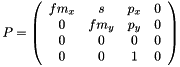Please note, that OpenGL's definition of the projection matrix looks different. OpenGL uses a flipped y-axis, and it's definition of projection also contains entries in the 3rd row. In our case, z values are not needed after the projection.

The cameras coordinate system transformation matrix C is defined by: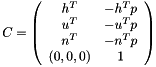Together, P and C are used to describe the projection model of the camera. A 3D utils::Point pw in the world is projected to the camera screen ps by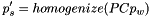ps contains just the first two components of p's. The homogenized operation devided a homogeneous 3D vector by it's 4th component.

In literature, sometimes are simgle 3x4 camera matrix is used. We call this matrix the camera's Q-matrix. The matrix contains all information that is neccessary for creating a camera. Usually, it can be decomposed into P and C using QR-decomposition. The icl::Camera class provides function to directly obtain a camera's Q-matrix. Q is defined by the first two and the last row of the matrix product P C.

## Camera Calibration

Valid camera instances can either be set up manually, or they can be created by camera calibration. ICL uses a quite simple yet very powerful calibration technique, which needs a set of at least 8 non-coplanar world points and their corresponding image coordinates. ICL features an easy to use camera calibration tool, which is discribed on ICL's website.

## ◆ Mat3x3

 typedef math::FixedMatrix icl::geom::Camera::Mat3x3

internal typedef

## ◆ Camera() [1/3]

 icl::geom::Camera::Camera ( const Vec & pos = Vec(0,0,10,1), const Vec & norm = Vec(0,0,-1,1), const Vec & up = Vec(1,0,0,1), float f = 3, const utils::Point32f & principalPointOffset = utils::Point32f(320,240), float sampling_res_x = 200, float sampling_res_y = 200, float skew = 0, const RenderParams & renderParams = RenderParams() )
inline

basic constructor that gets all possible parameters

## ◆ Camera() [2/3]

 icl::geom::Camera::Camera ( const std::string & filename, const std::string & prefix = "config." )

loads a camera from given file

Parameters
 filename file name of valid configuration file (in ICL's ConfigFile core::format) prefix valid prefix that determines wheret to find the camera within the given config file (note, that this prefix must end with '.')

## ◆ Camera() [3/3]

 icl::geom::Camera::Camera ( std::istream & configDataStream, const std::string & prefix = "config." )

loads a camera from given input stream

Parameters
 configDataStream stream object to read and interpret input file name of valid configuration file (in ICL's ConfigFile core::format) prefix valid prefix that determines where to find the camera within the given config file (note, that this prefix must end with '.')

## ◆ calibrate()

 static Camera icl::geom::Camera::calibrate ( std::vector< Vec > Xws, std::vector< utils::Point32f > xis, float focalLength = 1, bool performLMAOptimization = true )
static

Uses the passed world point – image point references to estimate the projection parameters.

At least 6 data points references are needed. It is not possible to estimate the focal length f directly, but only the products f*m_x and f*m_y (which is sufficient for defining the projection). Therefore an arbitrary value for f != 0 may be passed to the function. The method minimizes the algebraic error with the direct linear transform algorithm in which an SVD is used.

## ◆ calibrate_extrinsic() [1/3]

 static Camera icl::geom::Camera::calibrate_extrinsic ( const std::vector< Vec > & Xws, const std::vector< utils::Point32f > & xis, const Camera & intrinsicCamValue, const RenderParams & renderParams = RenderParams(), bool performLMAOptimization = true )
static

performs extrinsic camera calibration using a given set of 2D-3D correspondences and the given intrinsic camera calibration data

Camera::calibrate_extrinsic((std::vector<Vec>,std::vector<utils::Point32f>,float,float,float,float,float)

## ◆ calibrate_extrinsic() [2/3]

 static Camera icl::geom::Camera::calibrate_extrinsic ( const std::vector< Vec > & Xws, const std::vector< utils::Point32f > & xis, const Mat & camIntrinsicProjectionMatrix, const RenderParams & renderParams = RenderParams(), bool performLMAOptimization = true )
static

performs extrinsic camera calibration using a given set of 2D-3D correspondences and the given intrinsic camera calibration data

Camera::calibrate_extrinsic((std::vector<Vec>,std::vector<utils::Point32f>,float,float,float,float,float)

## ◆ calibrate_extrinsic() [3/3]

 static Camera icl::geom::Camera::calibrate_extrinsic ( std::vector< Vec > Xws, std::vector< utils::Point32f > xis, float fx, float fy, float s, float px, float py, const RenderParams & renderParams = RenderParams(), bool performLMAOptimization = true )
static

performs extrinsic camera calibration using a given set of 2D-3D correspondences and the given intrinsic camera calibration data

In many cases, when camera calibration is performed in a realy scene, it is quite difficult to place the calibration object well alligned and still in such a way that it covers a major fraction of the camera image. Instead, the calibration object usually is rather small, which leads to a poor calibration performace.

Tests showed (here, we used rendered images of a calibration object to get real ground-truth data) that in case of the common calibration performed by Camera::calibrate or Camera::calibrate_pinv sometimes lead to extreme camera positioning errors when the calibration object is too far away. The reason for this is that a far-awys calibration object looks more and more isometric in the camera image which makes it more and more difficult for the method to distinguish between a closer camera with a shot focal length or a futher-away camera with a higher focal length. Unfortunately, this effect often seems to be optimized by favoring one or another of these quantities, so even z-positioning errors of more than 10 cm could be observed. This effect is less important, when using such a camera in a multi-camera setup, as here, the error that is introduced is similarly smally as the missing-vanishing-point-effect that caused it in the first place. However, in other applications or even when calibrating a Kinect-Device, the camera position defines the basis for point cloud creation, so here a better positioning is mandatory.

To avoid these issues, it is recommended to perform the camera calibration in two steps. In the first step, only the intrinsic camera parameters are optimized. During this step, camera and calibration object can be positioned arbitrarily so that the calibration object perfectly covers the whole image space. In the 2nd step, the already obtained intrinsic parameters are fixed so that only the camera's extrinsic parameters (position and orientation) has to be optimized

# Algorithms

Outgoing from the a the camera's projection law: (u',v', 0, h) = P C x, which results in homogenized real screen coordinates u = u'/h and v = v'/h the method internally creates a system of linear equation to get a least square algebraic optimum.

Letbe the projection matrix and C the camera's coordinate frame transformation matrix (which transforms points from the world frame into the local camera frame. Further, we denote the lines of C by C1, C2, C3 and C4.

The projection law leads to the two formulas for u and v: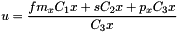and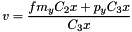which can be made linear wrt. the coefficients of C by deviding by C3x and then bringing the left part to the right, yielding: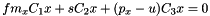and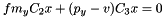This allows us to create a linear system of equations of the shape Ax=0, which is solved by finding the eigenvector to the smallest eigenvalue of A (which is internally done using SVD).

For each input point x=(x,y,z,1) and corresponding image point (u,v), we define du = px - u and dv = py - v in order to get two lines of the equation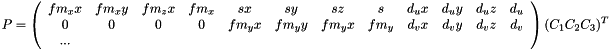.

Solving Px=0, yields a 12-D vectors whose elements written row-by-row into the first 3 lines of a 4x4 identity matrix is almost our desired relative camera transform matrix C. Actually, we receive only a scaled version of the solution C' = kC, which has to be normalized to make the rotation part become unitary. The normalization is performed using RQ-decomposition on the rotation part R' (upper left 3x3 sub-matrix of C'), which decomposes R' into a product R'=R*Q. Here, per definition, the resulting Q is unitary and therefore it is identical to the actual desired rotation part of C. As the RQ-decomposition does two things at once, it normalizes and it orthogonalizes the rows and colums of R', the factor k that is needed to also scale the translation part of C' cannot simply be extracted using e.g. R'(0,0) / Q(0,0) or a mean fraction between corresponding elements. Instead, we use the trace(R)/3, which could be shown to provide better results.

# Further non-linear optimization

Optionally, the resulting camera parameters, which result from an algebraic error minimization, can be optimized wrt. the pixel projection error. Experiments showed, that this can reduce the actual error by a factor of 10. The LMA-based optimization takes slightly longer then the normal linear optimization, but it is still real-time applicable and it should not increase the error, which is why, it is recommended to be used!

# The function parameters

fx, fy are the known camera x- and y-focal lengths, s is the skew, and px and py is the principal point offset

## ◆ calibrate_pinv()

 static Camera icl::geom::Camera::calibrate_pinv ( std::vector< Vec > Xws, std::vector< utils::Point32f > xis, float focalLength = 1, bool performLMAOptimization = true )
static

Uses the passed world point – image point references to estimate the projection parameters.

Same as the method calibrate, but using a pseudoinvers instead of the SVD for the estimation. This method is less stable and less exact.

## ◆ checkAndFixPoints()

 static void icl::geom::Camera::checkAndFixPoints ( std::vector< Vec > & worldPoints, std::vector< utils::Point32f > & imagePoints )
staticprivate

internally used utility function

## ◆ create_camera_from_calibration_or_udist_file()

 static Camera icl::geom::Camera::create_camera_from_calibration_or_udist_file ( const std::string & filename )
static

parses a given image-undistortion file and creates a camera

Please note that only the camera's intrinsic parameters are in the file, so extrinsic parameters for position and orientation will be identical to a defautl created Camera instance. The undistortion file must use the model "MatlabModel5Params". The given resolution is not used if the given file is a standard camera-calibration file

## ◆ createFromProjectionMatrix()

 static Camera icl::geom::Camera::createFromProjectionMatrix ( const math::FixedMatrix< icl32f, 4, 3 > & Q, float focalLength = 1 )
static

Compute all camera parameters from the 4x3 projection matrix.

## ◆ createTransformationMatrix()

 static Mat icl::geom::Camera::createTransformationMatrix ( const Vec & norm, const Vec & up, const Vec & pos )
staticprotected

## ◆ estimate3DPosition()

 Vec icl::geom::Camera::estimate3DPosition ( const utils::Point32f & pixel, const PlaneEquation & plane ) const

returns estimated 3D point for given pixel and plane equation

## ◆ estimate_3D()

 static Vec icl::geom::Camera::estimate_3D ( const std::vector< Camera * > cams, const std::vector< utils::Point32f > & UVs, bool removeInvalidPoints = false )
static

computes the 3D position of a n view from n cameras

Parameters
 cams list of cameras UVs list of image points (in image coordinates) removeInvalidPoints if this flag is set to true, all given points are checked to be within the cameras viewport. If not, these points are removed internally.

### Method:

This function uses a standard linear approach using a pseudo-inverse to solve the problem in a "least-square"-manner:

The camera is essentially represented by the 3x4-Q-Matrix, which can be obtained using icl::Camera::getQMatrix() const. Q is defined as follows:

            | -- x --  tx |
Q = [R|t] = | -- y --  ty |
| -- z --  tz |


The camera projection is trivial now. For a given projected point [u,v]' (in image coordinates) and a position in the world Pw (homogeneous):

[u,v,1*]' = hom( Q Pw )


Where '1*' becomes a real 1.0 just because of the homogenization step using hom(). Component-wise, this can be re-written as:

u  = x Pw + tx
v  = y Pw + ty
1* = z Pw + tz


In order to ensure, '1*' becomes a real 1.0, the upper two equations have to be devided by (z Pw + tz), which provides us the following two equations:

u = (x Pw + tx) / (z Pw + tz)
v = (y Pw + ty) / (z Pw + tz)


These equations have to be reorganized so that Pw can be factored out:

(u z - x) Pw = tx - u tz
(v z - y) Pw = ty - v tz


Now we can write this in matrix notation again:

A Pw = B      ,where
A = | u z - x |
| v z - y |
B = | tx - u tz |
| ty - v tz |

The obviously under-determined equation-system above uses only a single camera. If we put the results from several views together into a single equation system, it becomes unambigoulsly solvable using a pseudo-inverse approach:

| A1 |      | B1 |             | A1 |+ | B1 |
| A2 | Pw = | B2 |    => Pw =  | A2 |  | B2 |
|....|      |....|             |....|  |....|


where 'A+' means the pseudo-inverse of A

## ◆ estimate_3D_svd()

 static Vec icl::geom::Camera::estimate_3D_svd ( const std::vector< Camera * > cams, const std::vector< utils::Point32f > & UVs )
static

multiview 3D point estimation using svd-based linear optimization (should not be used)

This functions seems to provide false results for more than 2 views: use estimate_3D instead

## ◆ estimatePose()

 Mat icl::geom::Camera::estimatePose ( const std::vector< Vec > & objectCoords, const std::vector< utils::Point32f > & imageCoords, bool performLMAOptimization = true )

estimate world frame pose of object specified by given object points

## ◆ getAllViewRays()

 utils::Array2D icl::geom::Camera::getAllViewRays ( ) const

returns a 2D array of all viewrays

This method is much faster than using getViewRay several times since the projection matrix inversion that is necessary must only be done once

## ◆ getCSTransformationMatrix()

 Mat icl::geom::Camera::getCSTransformationMatrix ( ) const

get world to image coordinate system transformation matrix

## ◆ getCSTransformationMatrixGL()

 Mat icl::geom::Camera::getCSTransformationMatrixGL ( ) const

get world to image coordinate system transformation matrix

## ◆ getFocalLength()

 float icl::geom::Camera::getFocalLength ( ) const
inline

## ◆ getHoriz()

 Vec icl::geom::Camera::getHoriz ( ) const
inline

## ◆ getIntersection()

 static Vec icl::geom::Camera::getIntersection ( const ViewRay & v, const PlaneEquation & plane )
static

calculates the intersection point between this view ray and a given plane

Throws an utils::ICLException in case of parallel plane and line A ViewRay is defined by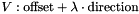A Plane is given by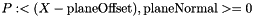Intersection is described by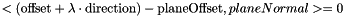which yields: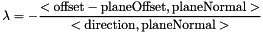and .. obviously, we get no intersection if direction is parallel to planeNormal

## ◆ getInvCSTransformationMatrix()

 Mat icl::geom::Camera::getInvCSTransformationMatrix ( ) const

returns the transform of the camera wrt. world frame

## ◆ getInvQMatrix()

 math::FixedMatrix icl::geom::Camera::getInvQMatrix ( ) const

returns the inverse QMatrix

## ◆ getName()

 const std::string& icl::geom::Camera::getName ( ) const
inline

## ◆ getNorm()

 const Vec& icl::geom::Camera::getNorm ( ) const
inline

## ◆ getPosition()

 const Vec& icl::geom::Camera::getPosition ( ) const
inline

## ◆ getPrincipalPointOffset()

 utils::Point32f icl::geom::Camera::getPrincipalPointOffset ( ) const
inline

## ◆ getPrincipalPointOffsetX()

 float icl::geom::Camera::getPrincipalPointOffsetX ( ) const
inline

## ◆ getPrincipalPointOffsetY()

 float icl::geom::Camera::getPrincipalPointOffsetY ( ) const
inline

## ◆ getProjectionMatrix()

 Mat icl::geom::Camera::getProjectionMatrix ( ) const

get projection matrix

## ◆ getProjectionMatrixGL()

 Mat icl::geom::Camera::getProjectionMatrixGL ( ) const

get the projection matrix as expected by OpenGL

## ◆ getQMatrix()

 math::FixedMatrix icl::geom::Camera::getQMatrix ( ) const

returns the common 4x3 camera matrix

## ◆ getRenderParams() [1/2]

 const RenderParams& icl::geom::Camera::getRenderParams ( ) const
inline

## ◆ getRenderParams() [2/2]

 RenderParams& icl::geom::Camera::getRenderParams ( )
inline

## ◆ getResolution()

 const utils::Size& icl::geom::Camera::getResolution ( ) const
inline

returns the current chipSize (camera resolution in pixels)

## ◆ getSamplingResolutionX()

 float icl::geom::Camera::getSamplingResolutionX ( ) const
inline

## ◆ getSamplingResolutionY()

 float icl::geom::Camera::getSamplingResolutionY ( ) const
inline

## ◆ getSkew()

 float icl::geom::Camera::getSkew ( ) const
inline

## ◆ getUp()

 const Vec& icl::geom::Camera::getUp ( ) const
inline

## ◆ getViewportMatrixGL()

 Mat icl::geom::Camera::getViewportMatrixGL ( ) const

## ◆ getViewRay() [1/2]

 ViewRay icl::geom::Camera::getViewRay ( const utils::Point32f & pixel ) const

Returns a view-ray equation of given pixel location.

## ◆ getViewRay() [2/2]

 ViewRay icl::geom::Camera::getViewRay ( const Vec & Xw ) const

Returns a view-ray equation of given point in the world.

## ◆ getViewRays()

 std::vector icl::geom::Camera::getViewRays ( const std::vector< utils::Point32f > & pixels ) const

returns a list of viewrays corresponding to a given set of pixels

This method is much faster than using getViewRay several times since the projection matrix inversion that is necessary must only be done once

 static void icl::geom::Camera::load_camera_from_stream ( std::istream & is, const std::string & prefix, Camera & cam )
staticprivate

intenal helper function

## ◆ optimize_camera_calibration_lma()

 static Camera icl::geom::Camera::optimize_camera_calibration_lma ( const std::vector< Vec > & Xws, const std::vector< utils::Point32f > xis, const Camera & init )
static

performs a non-linear LMA-based optimization to improve camera calibration results

## ◆ orthogonalizeRotationMatrix()

 void icl::geom::Camera::orthogonalizeRotationMatrix ( )

extracts the current rotation matrix and uses gramSchmidth orthogonalization

## ◆ project() [1/3]

 utils::Point32f icl::geom::Camera::project ( const Vec & Xw ) const

Project a world point onto the image plane. Caution: Set last component of world points to 1.

## ◆ project() [2/3]

 void icl::geom::Camera::project ( const std::vector< Vec > & Xws, std::vector< utils::Point32f > & dst ) const

Project a vector of world points onto the image plane. Caution: Set last component of world points to 1.

## ◆ project() [3/3]

 const std::vector icl::geom::Camera::project ( const std::vector< Vec > & Xws ) const

Project a vector of world points onto the image plane. Caution: Set last component of world points to 1.

## ◆ projectGL() [1/3]

 Vec icl::geom::Camera::projectGL ( const Vec & Xw ) const

Project a world point onto the image plane.

## ◆ projectGL() [2/3]

 void icl::geom::Camera::projectGL ( const std::vector< Vec > & Xws, std::vector< Vec > & dst ) const

Project a vector of world points onto the image plane.

## ◆ projectGL() [3/3]

 const std::vector icl::geom::Camera::projectGL ( const std::vector< Vec > & Xws ) const

Project a vector of world points onto the image plane.

## ◆ setFocalLength()

 void icl::geom::Camera::setFocalLength ( float value )
inline

## ◆ setName()

 void icl::geom::Camera::setName ( const std::string & name )
inline

## ◆ setNorm()

 void icl::geom::Camera::setNorm ( const Vec & norm, bool autoOrthogonalizeRotationMatrix = false )
inline

## ◆ setPosition()

 void icl::geom::Camera::setPosition ( const Vec & pos )
inline

## ◆ setPrincipalPointOffset() [1/2]

 void icl::geom::Camera::setPrincipalPointOffset ( float px, float py )
inline

## ◆ setPrincipalPointOffset() [2/2]

 void icl::geom::Camera::setPrincipalPointOffset ( const utils::Point32f & p )
inline

## ◆ setRenderParams()

 void icl::geom::Camera::setRenderParams ( const RenderParams & rp )
inline

## ◆ setResolution() [1/2]

 void icl::geom::Camera::setResolution ( const utils::Size & newScreenSize )

Changes the camera resolution and adapts dependent values.

Internally, this function also adapts the render parameters chipSize and viewport Furthermore, the prinizipal-point-offset is automatically set to the center of the new screen

## ◆ setResolution() [2/2]

 void icl::geom::Camera::setResolution ( const utils::Size & newScreenSize, const utils::Point & newPrincipalPointOffset )

Changes the camera resolution and adapts dependent values.

Internally, this function also adapts the render parameters chipSize and viewport Furthermore, the prinizipal-point-offset is set to the new given value

## ◆ setRotation() [1/2]

 void icl::geom::Camera::setRotation ( const Mat3x3 & rot )

set the norm and up vectors according to the passed rotation matrix

## ◆ setRotation() [2/2]

 void icl::geom::Camera::setRotation ( const Vec & rot )

set norm and up vectors according to the passed yaw, pitch and roll

## ◆ setSamplingResolution()

 void icl::geom::Camera::setSamplingResolution ( float x, float y )
inline

## ◆ setSamplingResolutionX()

 void icl::geom::Camera::setSamplingResolutionX ( float value )
inline

## ◆ setSamplingResolutionY()

 void icl::geom::Camera::setSamplingResolutionY ( float value )
inline

## ◆ setSkew()

 void icl::geom::Camera::setSkew ( float value )
inline

## ◆ setTransformation()

 void icl::geom::Camera::setTransformation ( const Mat & m )

sets the camera's rotation and position from given 4x4 homogeneous matrix

Note, that this function is designed to not change the camera when calling cam.setTransfromation(cam.getCSTrannsformationMatrix()). In other words, this means, that it will use the given rotation part R, as rotation matrix, but set the camera position to -R*t

## ◆ setUp()

 void icl::geom::Camera::setUp ( const Vec & up, bool autoOrthogonalizeRotationMatrix = false )
inline

gets automatically normalized

## ◆ setWorldFrame()

 void icl::geom::Camera::setWorldFrame ( const Mat & m )

adapts the camera transformation, so that the given frame becomes the world frame

To this end, the camera's world transform is set to m.inv() * cam.getInvCSTransformationMatrix()

## ◆ setWorldTransformation()

 void icl::geom::Camera::setWorldTransformation ( const Mat & m )

this sets the camera to the given world tranformation

If m is [R|t], the function sets the camera rotation to R and the position to t. Note that getRotation() will return R^-1 which is R^t

## ◆ toString()

 std::string icl::geom::Camera::toString ( ) const

## ◆ translate()

 void icl::geom::Camera::translate ( const Vec & d )
inline

translates the current position vector

## ◆ m_f

 float icl::geom::Camera::m_f
private

focal length

## ◆ m_mx

 float icl::geom::Camera::m_mx
private

## ◆ m_my

 float icl::geom::Camera::m_my
private

sampling resolution

## ◆ m_name

 std::string icl::geom::Camera::m_name
private

name of the camera (visualized in the scene if set)

## ◆ m_norm

 Vec icl::geom::Camera::m_norm
private

normal vector of image plane

## ◆ m_pos

 Vec icl::geom::Camera::m_pos
private

center position vector

## ◆ m_px

 float icl::geom::Camera::m_px
private

## ◆ m_py

 float icl::geom::Camera::m_py
private

principal point offset

## ◆ m_renderParams

 RenderParams icl::geom::Camera::m_renderParams
private

## ◆ m_skew

 float icl::geom::Camera::m_skew
private

skew parameter in the camera projection, should be zero

## ◆ m_up

 Vec icl::geom::Camera::m_up
private

vector pointing to pos. y axis on image plane

The documentation for this class was generated from the following file:
• /Users/alneuman/vm/icl/ICLGeom/src/ICLGeom/Camera.h